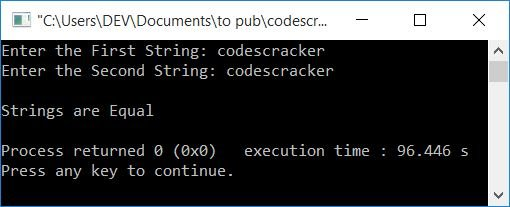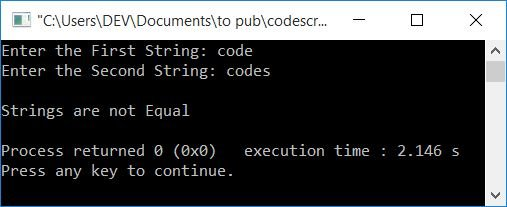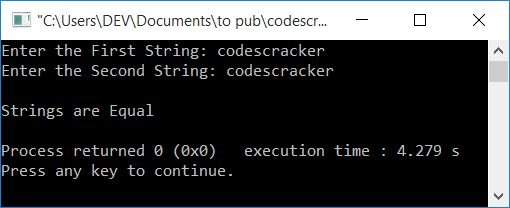# C++ program to compare two strings

This article will teach you how to compare two strings in C++ and provide you with code to do so. The program is created in the following ways:

## Without using strcmp(), compare two strings

To compare two strings in C++ programming, you have to ask the user to enter the two strings and compare them without using any type of library function like strcmp(), as shown in the program given below. Let's have a look at the program first; we'll get the explanation later on.

```#include<iostream>
using namespace std;
int main()
{
char str1, str2;
int i=0, chk=0;
cout<<"Enter the First String: ";
cin>>str1;
cout<<"Enter the Second String: ";
cin>>str2;
while(str1[i]!='\0' || str2[i]!='\0')
{
if(str1[i]!=str2[i])
{
chk = 1;
break;
}
i++;
}
if(chk==0)
cout<<"\nStrings are Equal";
else
cout<<"\nStrings are not Equal";
cout<<endl;
return 0;
}```

This program was built and runs under the Code::Blocks IDE. Here is its sample run:Now enter the value of the first string, "codescracker," and then again enter the value of the second string, "codescracker." Press the ENTER key to see the output as shown in the snapshot given below:Here is another sample run, with user input codescracker as the first string and crackercodes as the second string:And here is the last sample run with the user input code as the first string and codes as the second input:When the user enters the first string, then it gets initialized to str1 in a way that, supposing the user enters code as the first string:

• The first character (c) gets initialized to str1.
• The second character (o) gets initialized to str1.
• Likewise, str1=d, str1=e.
• Then a null-terminated character \0 is automatically assigned after the last character of the entered string, so str=\0.

In a similar way, the second string gets initialized to str2. Now with user input, code, and codes as the first and second strings, the dry run of the above program goes like this:

• Inside the while loop, the condition is:
`str1[i]!='\0' || str2[i]!='\0'`
Because i = 0 at first, the condition changes after i is replaced with 0.
`str1!='\0' || str2!='\0'`
On putting the character present at the 0th index of both the strings.
`c!='\0' || c!='\0'`
then the condition is evaluated to be true.
• Because neither the first or second string's first character is equal to the null-terminated character \0.
• Therefore, program flow goes inside the loop and evaluates the condition of the if statement, that is,
`str1[i]!=str2[i]`
or
`c!=c`
This evaluates to false. Therefore, program flow does not go inside the if's body, rather increments the value of i and goes back to the condition of the while loop again.
• Because i=1 now, repeat the process with the updated value of i.
• PThe process is repeated until one of the two conditions within the while loop evaluates to false. Or if the condition of if (inside the loop) evaluates to be true, therefore program flow goes inside the if's body and initializes 1 to chk, then using the break keyword, program flow quits the while loop for further execution.
• After exiting from the while loop (either by evaluating its condition as false, or by evaluating the if's condition as true), check for the value of chk in such a way that, if it holds its initial value of 0, then program flow never goes inside the if's body. This means that no characters in either string at the same index are mismatched. It means that strings are equal. Otherwise, if any mismatches are found, then strings are not equal.

### What if the user enters strings of unequal length?

As shown in the output with two strings, code and code, the while loop runs four times without reason (comparing code, the first four characters of the first string, with codes, the first four characters of the second string).Because if the lengths of two strings are not equal, then they surely cannot be the same.

So we do not need to evaluate while looping in that case. Therefore, the program given below first finds the length of each string and then checks whether the length of both strings is equal or not. If it is equal, then we will proceed to compare the strings. Otherwise, print a message like "unequal length" or anything else (depends on you):

```#include<iostream>
using namespace std;
int main()
{
char str1, str2;
int i, chk=0, len1=0, len2=0;
cout<<"Enter the First String: ";
cin>>str1;
cout<<"Enter the Second String: ";
cin>>str2;
i=0;
while(str1[i]!='\0')
{
len1++;
i++;
}
i=0;
while(str2[i]!='\0')
{
len2++;
i++;
}
if(len1==len2)
{
i=0;
while(str1[i]!='\0' || str2[i]!='\0')
{
if(str1[i]!=str2[i])
{
chk = 1;
break;
}
i++;
}
if(chk==0)
cout<<"\nStrings are Equal";
else
cout<<"\nStrings are not Equal";
}
else
cout<<"\nString Length must has to be same, to Compare!";
cout<<endl;
return 0;
}```

Here's an example of how it works with user input code and codes as first and second strings:## Compare two strings using strcmp()

With the help of library functions, the program becomes smaller. We don't need to write any extra code to perform tasks like finding the length of a string or comparing strings; library functions like strlen() and strcmp() can handle these tasks easily.

The function strcmp() takes two strings as arguments and returns 0 if both strings are equal. And the function strlen() takes a single string as an argument and returns its length.

```#include<iostream>
#include<string.h>
using namespace std;
int main()
{
char str1, str2;
int len1, len2;
cout<<"Enter the First String: ";
cin>>str1;
cout<<"Enter the Second String: ";
cin>>str2;
len1 = strlen(str1);
len2 = strlen(str2);
if(len1==len2)
{
if(strcmp(str1, str2)==0)
cout<<"\nStrings are Equal";
else
cout<<"\nStrings are not Equal";
}
else
cout<<"\nStrings are not Equal";
cout<<endl;
return 0;
}```

Here's a sample run with user input codescracker as both the first and second strings:#### The same program in different languages

C++ Quiz

« Previous Program Next Program »